# Orchard

10 trees in 5 lines grows in the orchard. How many trees are in the orchard?

x =  50

### Step-by-step explanation: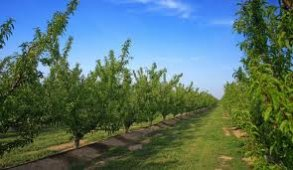Did you find an error or inaccuracy? Feel free to write us. Thank you!Math student
I which you guys let us type math problemsMath student
Trees in a 3 by 3 configuration make 6 lines, 3 top to bottom lines and 3 left to right.  3 trees in 1 line plus 3 trees in a perpendicular line and 3 trees in another perpendicular line not shadowing the first are 7 treesTips to related online calculators
Would you like to compute count of combinations?

## Related math problems and questions:

• Orchard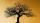3/5 of the trees in the orchard are apples, 1/3 are cherries and the remaining five trees are pear. How many trees are in the orchard?
• Trees - orchardThe 3/5 trees are apple trees and 1/3 of the trees are cherries. The remaining 5 trees are pear trees. How many trees are in the orchard.
• Orchard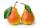Three-fifths of the orchard are apple trees, one-third of the trees are cherries. The remaining 5 trees are pears. How many trees are in the orchard?
• Draw it!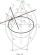Draw two lines c, d that c || d. On line c, mark the points A, B. By point A, a lead perpendicular line to c. By point B, lead perpendicular line to c.
• DigitsHow many odd four-digit numbers can we create from digits: 0, 3, 5, 6, 7? (a) the figures may be repeated (b) the digits may not be repeated
• Expression plus minusEvaluate expression: (-1)2 . 12 – 6 : 3 + (-3) . (-2) + 22 – (-3) . 2
• Kangaroo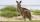Kangaroo does 10 jumps in 1 minute and then three minutes lie, then will make 10 jumps in 1 minute and 3 minutes lie, and so on. Find the shortest time in which a kangaroo does 30 jumps.
• Bucket of clay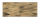Tina and Bill share a 12-ounce bucket of clay. By the end of the week, Tina has used 1/6 of the bucket, and Bill has used 2/3 of the bucket of clay. How many ounces are left in the bucket?
• Neighborhood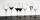I have 7 cups: 1 2 3 4 5 6 7. How many opportunities of standings cups are there if 1 and 2 are always neighborhood?
• SalamiHow many ways can we choose 5 pcs of salami if we have 6 types of salami for 10 pieces and one type for 4 pieces?
• Trees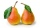Two-fifths of the trees in the orchard are apple trees, one-sixth a pear. The remaining 26 trees are cherries. How many pears are in the orchard?
• Logik game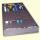Letter game Logik is a two player game, which has the following rules: 1. The first player thinks five-letter word in which no letter is not repeated. 2. The second player writes a five-letter word. 3. The first player answers two numbers - the first numb
• Forest ranger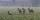Forest ranger watching from the hide deers. At one time point, he can see six deers. How many maximum deers can be seen by three rangers at the same time?
• Trees3/5 trees are apples, cherries are 1/3. 5 trees are pear. How many is the total number of trees?
• Straight lines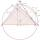Draw two lines c, d so that c || d. On line c mark points A, B, from point A start perpendicular to line c, from point B perpendicular to line c.
• Math classificationIn 3A class are 27 students. One-third got a B in math, and the rest got an A. How many students received a B in math?
• Evaluate 11Multiply the quotient of 6 and 2 by 3, then add 1. Add 1 and 6, then divide by 2 and multiply by 3. Divide 6 by the product of 2 and 3, then add 1.